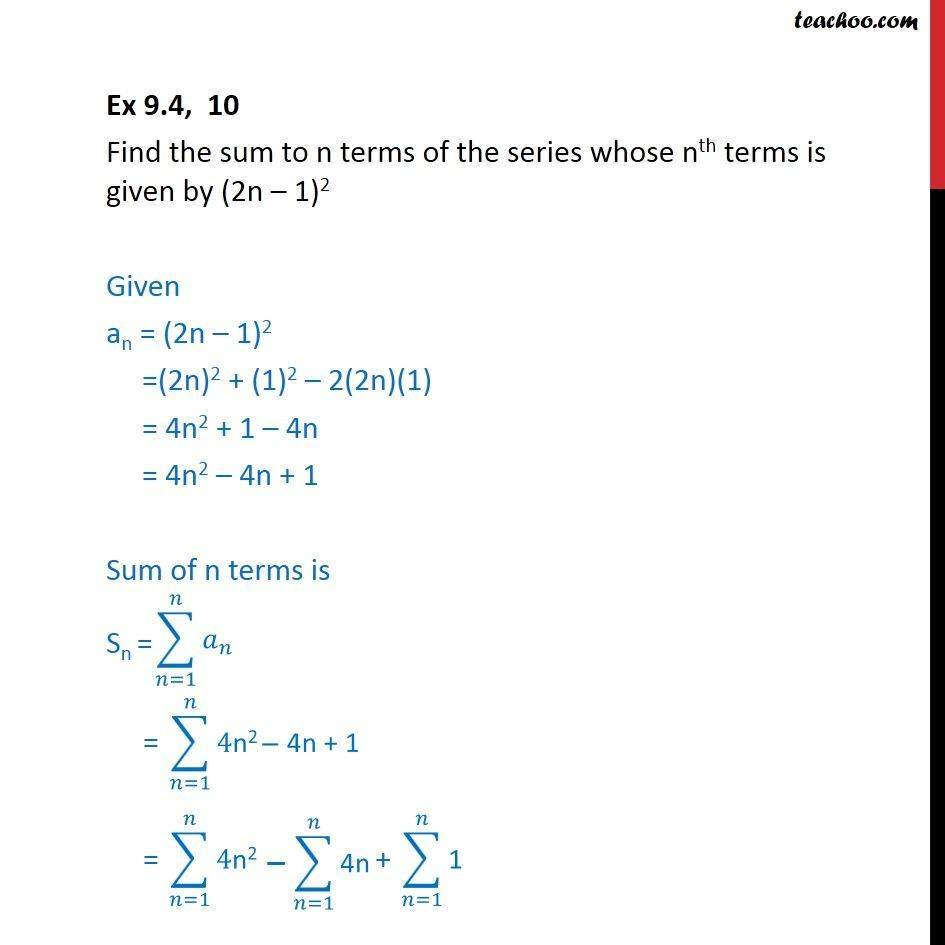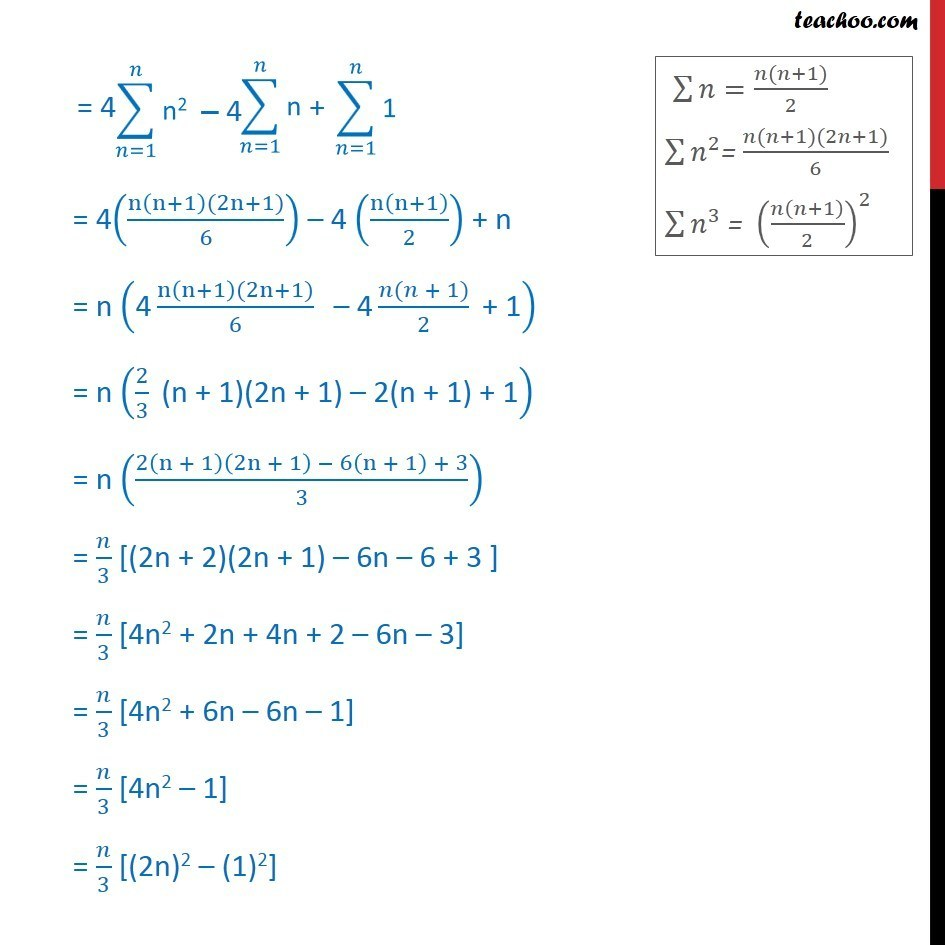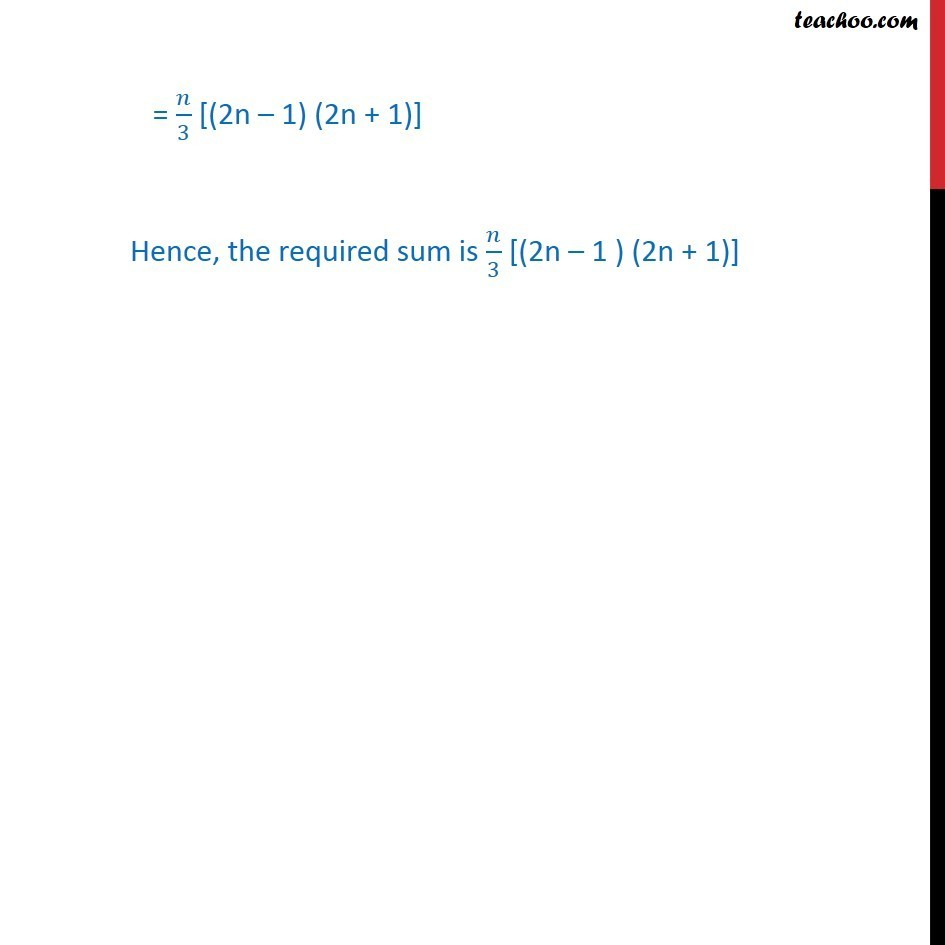Finding sum from nth number

Chapter 8 Class 11 Sequences and Series
Concept wiseLearn in your speed, with individual attention - Teachoo Maths 1-on-1 Class

### Transcript

Question 10 Find the sum to n terms of the series whose nth terms is given by (2n 1)2 Given an = (2n 1)2 =(2n)2 + (1)2 2(2n)(1) = 4n2 + 1 4n = 4n2 4n + 1 Sum of n terms is = 4((n(n+1)(2n+1))/6) 4 (n(n+1)/2) + n = n ("4" (n(n+1)(2n+1))/6 " 4" ( ( + 1))/2 " + 1" ) = n (2/3 " (n + 1)(2n + 1) 2(n + 1) + 1" ) = n ((2(n + 1)(2n + 1) 6(n + 1) + 3)/3) = /3 [(2n + 2)(2n + 1) 6n 6 + 3 ] = /3 [4n2 + 2n + 4n + 2 6n 3] = /3 [4n2 + 6n 6n 1] = /3 [4n2 1] = /3 [(2n)2 (1)2] = /3 [(2n 1) (2n + 1)] Hence, the required sum is /3 [(2n 1 ) (2n + 1)]